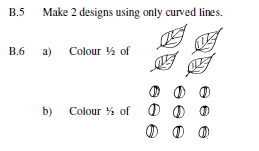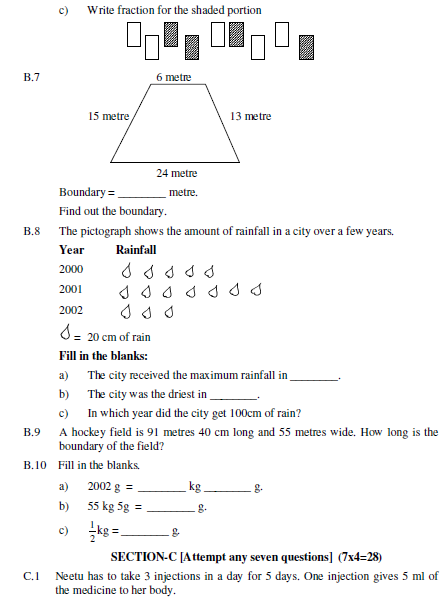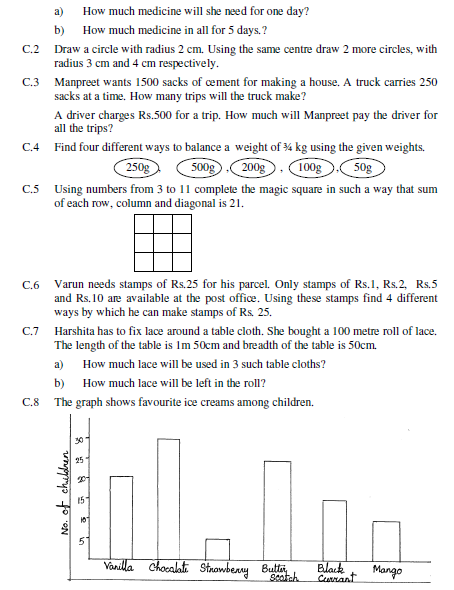# CBSE Class 4 Mathematics Sample Paper Set R

Read and download PDF of CBSE Class 4 Mathematics Sample Paper Set R designed as per the latest curriculum and examination pattern for Class 4 issued by CBSE, NCERT and KVS. The latest Class 4 Mathematics Sample Papers have been provided with solutions so that the students can solve these practice papers and then compare their answers. This will help them to identify mistakes and improvement areas in Mathematics Standard 4 which they need to study more to get better marks in Grade 4 exams. After solving these guess papers also refer to solved Class 4 Mathematics Question Papers available on our website to build strong understanding of the subject

## Sample Paper for Class 4 Mathematics Pdf

Students can refer to the below Class 4 Mathematics Sample Paper designed to help students understand the pattern of questions that will be asked in Grade 4 exams. Please download CBSE Class 4 Mathematics Sample Paper Set R

### Mathematics Class 4 Sample Paper

CBSE Class 4 Mathematics Sample Paper Set R. Sample Papers are the very important for every student. The sample papers should be practiced to gain extra marks in examinations. The sample papers have been prepared based on summative assessment1 and summative assessment 2 pattern. The sample papers have been prepared based on pattern of last year examinations and as per latest changes in the syllabus. Students, teachers and parents can download all CBSE educational material and very well prepared worksheets from this website.  All CBSE educational material is developed by our panel of teachers, have also been submitted by CBSE teachers and students.

Section -A

A.1 Standard unit of capacity is ______________.

A.2 ______________ of the circle is the longest chord of the circle.

A.3 4 × ______________make a whole.

A.4 9AB, 8 CD, 7EF, ______________, ______________

A.5 1234 × 1000 = ______________

A.6 A toffee weighs ______________

a. 500 gm b. 25 gm c. 1000 gm d. 2500 gm

A.7 Perimeter of a square is

a. side x side b. length x breadth c. 4×side d. 3×side

A.8 Pictorial representation of data is called.

a. Bargraph b. Smart Chart c. Pictograph d. Pie Chart

A.9 450 ml + ______ = 1l

a. 650 ml b. 550 ml c. 500 ml d. 450 ml

A.10 If radius = 12 cm then the diameter is

a. 24 cm b. 20 cm c. 36 cm d. 6 cm

A.11 ½ + ¼ + ______________ = 1 whole

a. ½ b. ⅓ c. ¼ d. ⅛

A.12 8000 ÷ 4 = ______________

a. 200 b. 2000 c. 20,000 d. 4000

A.13 333 ÷ 10 gives quotient

a. 30 b. 33 c. 330 d. 303

A.14 250 g + 250 g + ______________= 750 g

a. 500 g b. 250 g c. 550 g d. 50 g

A.15 If length of a rectangle is 11m and breadth is 7 m then perimeter of the rectangle is

a. 40 m b. 36 m c. 63 m d. 77 m

Write True or False.

A.16 We use symbols in pictograph.

A.17 If we join any two points on a circle we get a diameter

A.18 In fraction 89/100, numerator is 100.

A.19 One fourth is also called a quarter.

A.20 17235 g = 17 kg 235 g

SECTION-B

[Attempt any eight questions] (8x3=24)

B.1 There are 20 stars. A quarter of them are red. How many stars are red? How many are not red?

B.2 a) 8, 108, 208, ________, ________, ________, ________

b) 965, 865, 765, ________, ________, ________, ________

c) 28z, 27y, 26x, ________, ________, ________, ________

B.3 A frog jumps 3 steps at a time starting from o.

a) Count the jumps he takes to reach 27.

b) If he is at 42, he has taken .................... jumps.

B.4 a) 2× ________ ml =1l

b) 5 × ________ ml = 1l

c) 4×________ ml = 1lPlease click the below link to access CBSE Class 4 Mathematics Sample Paper Set R.